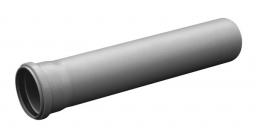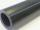# Plastic pipe

Calculate the plastic pipe's weight with diameter d = 70 mm and length 380 cm if the wall thickness is 4 mm and the density of plastic is 1367 kg/m3.

m =  4.3 kg

### Step-by-step explanation:Did you find an error or inaccuracy? Feel free to write us. Thank you!Tips to related online calculators
Do you know the volume and unit volume, and want to convert volume units?
Tip: Our Density units converter will help you with the conversion of density units.
Do you want to convert mass units?

#### You need to know the following knowledge to solve this word math problem:

We encourage you to watch this tutorial video on this math problem:

## Related math problems and questions:

• The squareThe square oak board (with density ρ = 700 kg/m3) has a side length of 50 cm and a thickness of 30 mm. 4 holes with a diameter of 40 mm are drilled into the board. What is the weight of the board?
• Iron densityCalculate the weight of a 2 m long rail pipe with an internal diameter of 10 cm and a wall thickness of 3 mm. The iron density is p = 7.8 g/cm3.
• Cu wireCopper wire has a length l = 820 m and diameter d = 10 mm. Calculate the weight if density of copper is ρ = 8500 kg/m3. Result round to one decimal place.
• Aluminum wireAluminum wire of 3 mm diameter has a total weight of 1909 kg and a density of 2700 kg/m3. How long is the wire bundle?
• Concrete hatchThe concrete hatch for a round well has a diameter of 1300 mm and a thickness of 80 mm. Determine its weight in kg if the density of the concrete is 2545 kg/m3
• 3d printer3D printing ABS filament with diameter 1.75 mm has density 1.04 g/cm3. Find the length of m = 5 kg spool filament. (how to calculate length)
• Wall thicknessThe hollow metal ball has an outside diameter of 40 cm. Determine the wall thickness if the weight is 25 kg and the metal density is 8.45 g/cm3.
• Oak trunkCalculate in tonnes the approximate weight of a cylindrical oak trunk with a diameter of 66 cm and a length of 4 m, knowing that the density of the wood was 800 kg/m³.
• Copper plateCalculate the thickness of the copper plate with a density 8.7 g/cm³ measuring 1.5 meters and 80 cm and its weight is 3.65 kg
• Hollow sphereCalculate the weight of a hollow stříbrné sphere (density 10.5 g/cm3) if the vnitřní diameter is 15 cm and wall thickness is 1 mm.
• Steel tubeThe steel tube has an inner diameter of 4 cm and an outer diameter of 4.8 cm. The density of the steel is 7800 kg/m3. Calculate its length if it weighs 15 kg.
• The coilHow many ropes (the diameter 8 mm) fit on the coil (threads are wrapped close together) The coil has dimension: the inner diameter 400mm, the outside diameter 800mm and the length of the coil is 470mm
• Iron sphereIron sphere has weight 100 kg and density ρ = 7600 kg/m3. Calculate the volume, surface, and diameter of the sphere.
• Cuboid 5Calculate the mass of the cuboid with dimensions of 12 cm; 0.8 dm and 100 mm made from spruce wood (density = 550 kg/m3).
• Brass tubeThe outer perimeter of brass tube (ρ = 8.5 g/cm3) is 38 cm. Its mass is 5 kg, length 54 cm. What is the pipe wall thickness?
• Copper Cu wireCopper wire with a diameter of 1 mm and a weight of 350 g is wound on a spool. Calculate its length if the copper density is p = 8.9 g/cm cubic.
• The pipeThe pipe is 1.5 m long. Its outer diameter is 60 cm, inner diameter is 52 cm. Calculate the pipe's weight if the material's density from which it is made is 2 g/cm3. Round the results to whole kilograms.